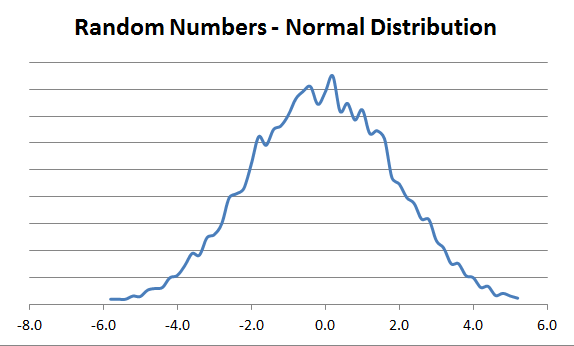## Excel Random Numbers

### Quickly And Easily Generate Pseudo-Random Numbers

This Excel file will give an example of how to generate random numbers in Excel.  You can generate integers or real numbers, and use those random numbers in a variety of ways such as sorting a list.  Combined with the NORM function, you can also generate random numbers in a normal distribution

50% Complete

You're Almost there! Please complete this form and click the button below to gain instant access.• Examples of random integers or real numbers, in different ranges
• Examples of using random numbers to sort a list
• Examples of generating random numbers in a normal curve distribution